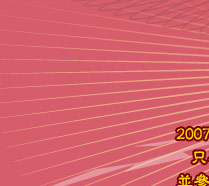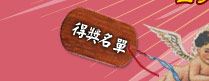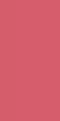<%@ codepage=65001 %> <% 'Response.CacheControl = "no-cache" 'Response.AddHeader "Pragma", "no-cache" Response.Expires = -1 Response.buffer = True %> <% pagesize = 50 thispage = 1 totalpages = 1 totalrecords = 1 page = trim(Request("page")) If page = "" Then page = "1" If Check_PartanValue(Global_validnum, page) = False Then page = "1" thispage = Clng(page) pc = trim(Request("pc")) pc = "33" ' 台客現場直擊為 33 If pc = "" Then pc = "0" If Check_PartanValue(Global_validnum, pc) = False Then pc = "0" wid = pc errcode = "" errMsg = "" HExhary = False Dim Exhary '投稿的陣列 Set fs = Server.CreateObject ("Scripting.FileSystemObject") Set rs = Server.CreateObject ("adodb.recordset") 'sql = "SELECT WID, WNAMETW, WNAMECN, " 'sql = sql & " (SELECT COUNT(*) AS Amt1 FROM tbl_p005 p5 INNER JOIN tbl_p004 p4 ON p5.WID = p4.WID INNER JOIN " 'sql = sql & " tbl_p003 p3 ON p5.SID = p3.SID INNER JOIN tbl_p001 p1 ON p3.PID = p1.PID INNER JOIN tbl_m003 m3 ON p1.MSID = m3.MSID INNER JOIN " 'sql = sql & " tbl_m001 m1 ON m3.MSID = m1.MSID WHERE (p5.Enabled = 1) AND (p3.PURL <> N'') AND (p3.Enabled = 1) AND " 'sql = sql & " (p4.Enabled = 1) AND (m1.Enabled = 1) AND (p1.Enabled = 1) AND (p4.WID = tbl_p004.wid)) AS Amt1 FROM tbl_p004 " 'sql = sql & " WHERE (Enabled = 1) AND (Online = 1) AND ((SELECT COUNT(*) AS Amt1 " 'sql = sql & " FROM tbl_p005 p5 INNER JOIN " 'sql = sql & " tbl_p004 p4 ON p5.WID = p4.WID INNER JOIN tbl_p003 p3 ON p5.SID = p3.SID INNER JOIN tbl_p001 p1 ON p3.PID = p1.PID INNER JOIN " 'sql = sql & " tbl_m003 m3 ON p1.MSID = m3.MSID INNER JOIN tbl_m001 m1 ON m3.MSID = m1.MSID WHERE (p5.Enabled = 1) AND (p3.PURL <> N'') AND (p3.Enabled = 1) " 'sql = sql & " AND (p4.Enabled = 1) AND (m1.Enabled = 1) AND (p1.Enabled = 1) AND (p4.WID = tbl_p004.wid)) > 0) ORDER BY ASORT, WID DESC" sql = "SELECT p5.WID, p4.WNAMETW" sql = sql & " FROM tbl_p001 p1 INNER JOIN tbl_p003 p3 ON p1.PID = p3.PID INNER JOIN tbl_p005 p5 INNER JOIN tbl_p004 p4 " sql = sql & " ON p5.WID = p4.WID ON p3.SID = p5.SID INNER JOIN tbl_m003 m3 ON p1.MSID = m3.MSID INNER JOIN tbl_m001 m1 ON m3.MSID = m1.MSID " sql = sql & " WHERE (p4.ASITE = '" & Global_AllocationSite & "') AND (m1.Enabled = 1) AND (p3.Enabled = 1) AND (p1.Enabled = 1) AND (p4.Enabled = 1) AND (p4.Online = 1) " sql = sql & " GROUP BY p5.WID, p4.WNAMETW, p4.ASORT ORDER BY p4.ASORT" hx = False Do rs.Open sql, conn, 3, 1 If rs.EOF Then Else HExhary = True Exhary = rs.GetRows () End If rs.Close If HExhary = False Then errMsg = "沒有投稿可以秀.....................啦啦啦......" Exit do End If For ij = 0 To Ubound(Exhary, 2) If Exhary(0, ij) & "" = wid Then WName = server.HTMLEncode (Exhary(1,ij)) hx = True Exit for End If Next If hx = False Then wid = Exhary(0,0) & "" WName = server.HTMLEncode (Exhary(1,0)) hx = True End If Exit Do Loop If errMsg = "" Then strHeaderLinks = " " & strHeaderSepaarate & " 相簿 " & strHeaderSepaarate & " 網友投稿 " & strHeaderSepaarate & " " & WName strPageTitle = "網友投稿 " & strTitleSepaarate & " " & WName End If %> <% Set rs = Nothing Set fs = Nothing Function main() If errMsg <> "" Then %> <% Exit function End If mainpage End Function Function mainpage() HRecs = False Dim RecAry ImgThumbMax = 90 na = "" surl = "index.asp?pc=" & wid ' & pc MaxCells = 5 'sql = "SELECT p5.WWID, p5.SID, p3.PSDESC, p3.TURL, p3.CCLICK, p5.CrDt, p1.PID, p1.PNAME, m1.MSID, m1.MNICKNAME " 'sql = sql & " FROM tbl_p005 p5 INNER JOIN tbl_p004 p4 ON p5.WID = p4.WID INNER JOIN " 'sql = sql & " tbl_p003 p3 ON p5.SID = p3.SID INNER JOIN tbl_p001 p1 ON p3.PID = p1.PID INNER JOIN " 'sql = sql & " tbl_m003 m3 ON p1.MSID = m3.MSID INNER JOIN tbl_m001 m1 ON m3.MSID = m1.MSID " 'sql = sql & " WHERE (p5.Enabled = 1) AND (p3.PURL <> N'') AND (p3.Enabled = 1) AND " 'sql = sql & " (p4.Enabled = 1) AND (m1.Enabled = 1) AND (p1.Enabled = 1) AND (p4.Online = 1) " 'sql = sql & " AND (p4.WID = " & wid & " ) " 'sql = sql & " ORDER BY p5.WWID DESC" 'sql = "SELECT p5.WWID, p3.TURL, p3.CCLICK, p5.CrDt" 'sql = sql & " FROM tbl_p005 p5 INNER JOIN tbl_p004 p4 ON p5.WID = p4.WID INNER JOIN tbl_p003 p3 ON p5.SID = p3.SID INNER JOIN" 'sql = sql & " tbl_p001 p1 ON p3.PID = p1.PID INNER JOIN tbl_m003 m3 ON p1.MSID = m3.MSID INNER JOIN tbl_m001 m1 ON m3.MSID = m1.MSID" 'sql = sql & " WHERE (p4.ASITE = '" & Global_AllocationSite & "') AND (p4.WID = " & wid & ") AND (p5.Enabled = 1) AND (p3.Enabled = 1) AND (p4.Enabled = 1) AND (m1.Enabled = 1) AND (p1.Enabled = 1) AND (p4.Online = 1) ORDER BY p5.WWID DESC" sql = "SELECT p5.WWID, p3.TURL, p3.CCLICK, p5.CrDt" sql = sql & " FROM tbl_m001 m1 INNER JOIN tbl_m003 m3 ON m1.MSID = m3.MSID INNER JOIN tbl_p005 p5 INNER JOIN tbl_p003 p3 ON p5.SID = p3.SID INNER JOIN tbl_p001 p1 ON p3.PID = p1.PID ON m1.MSID = p1.MSID " sql = sql & " WHERE (p5.WID = " & wid & ") AND (p5.Enabled = 1) AND (p3.Enabled = 1) AND (m1.Enabled = 1) AND (p1.Enabled = 1) ORDER BY p5.WWID DESC" rs.Open sql, conn, 3, 1 rs.PageSize = pagesize If rs.EOF Then totalrecords = 0 Else HRecs = True totalrecords = rs.RecordCount totalpages = rs.PageCount If thispage < 1 Then thispage = 1 If thispage > totalpages Then thispage = totalpages rs.AbsolutePage = thispage 'RecAry = rs.GetRows (pagesize) End If 'rs.Close howto = "5508" If Global_AllocationSite = "CN" Then howto = "28591" %><% If totalpages > 0 Then %> <% End If %>
<% If HRecs Then j = 0 Set Jpeg = Server.CreateObject("Persits.Jpeg") 'For ij = 0 To UBound(RecAry, 2) Do If rs.EOF Then Exit Do If j = pagesize Then Exit Do j = j + 1 'RecAry If j MOD MaxCells = 1 Then Response.Write "" End If %> <% 'If j MOD MaxCells = 0 AND ij < UBound(RecAry, 2) Then If j MOD MaxCells = 0 AND j < pagesize Then Response.Write "" End If rs.MoveNext Loop Set Jpeg = Nothing 'If j MOD MaxCells > 0 Then If j > 0 AND (j MOD MaxCells) > 0 Then For k = 1 To (MaxCells - (j MOD MaxCells)) Response.Write "" Next Response.Write "" End If Else %> <% End If rs.Close %>
 <% ' ff = trim(rs.Fields ("TURL").Value ) 'If NOT IsNULL(RecAry(3, ij)) Then ff = trim(RecAry(3, ij)) ' Response.Write getThumbPic(Jpeg, ImgThumbMax, ImgThumbMax, ff, na) %> " target="_blank">

<% BTNChangePage "index.asp?pc=" & wid & "&", totalpages, thispage, totalrecords, "", "p9-gray-content", "", "btn04", " 張" %>

<% End Function %>